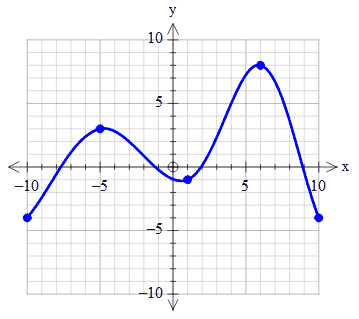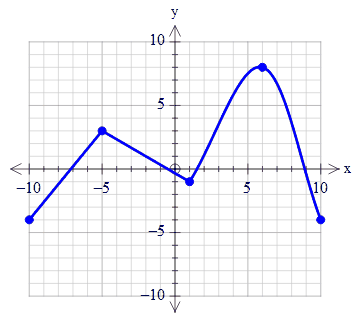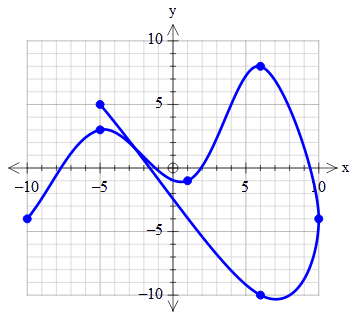﻿ Smoothed Curves

# Smoothed Curves

Top  Previous  Next
 If you join points with a tilde (~), the graph tool will draw a smoothed line joining the points.   For example, if you enter (-10,-4)~(-5,3)~(1,-1)~(6,8)~(10,-4) as your function, the graph tool will draw this graph.You can mix ~ and + in the same function.   (-10,-4)~(-5,3)+(1,-1)~(6,8)~(10,-4)The smoothed curves do not have to be left to right. In other words, they do not need to represent a function.   (-10,-4)~(-5,3)~(1,-1)~(6,8)~(10,-4)~(6,-10)~(-5,5)